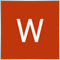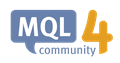# working with files and arrays81

hello

i have 2 arrays 1 to open files and 1 to delete it

but i have problem with the code

```int a = {11, 1, 3, 5, 17, 4, 2, 15};
int b = {1, 2, 3, 4, 5};
int filehandle;

for(int x=0; x<ArraySize(a); x++){
string n = IntegerToString(a[x]);
filehandle = FileOpen(n, FILE_WRITE|FILE_CSV);
FileClose(StrToInteger("n"));
}
for(int y=0; y<ArraySize(b); y++){
FileDelete(IntegerToString(b[y]));
} ```

the result should be 11, 17, 15 file name exist and the other files should be deleted

can anyone tell me whats wrong?151

```for(int x=0; x<ArraySize(a); x++){
string n = IntegerToString(a[x]);
filehandle = FileOpen(n, FILE_WRITE|FILE_CSV);
FileClose(filehandle);
}
for(int y=0; y<ArraySize(b); y++){
FileDelete(IntegerToString(b[y]));
} ```

StrToInteger("n") is wrong... you would need StrToInteger(n) to send the filename, but FileClose() needs filehandle not filename...151

https://docs.mql4.com/function_indicesList of MQL4 Functions - MQL4 Reference
• docs.mql4.com
Reads from the file of the CSV type a string of one of the formats: "YYYY.MM.DD HH:MM:SS", "YYYY.MM.DD" or "HH:MM:SS" - and converts it into a datetime value81